# RD Sharma Solutions for Class 6 Maths Chapter 6: Fractions Exercise 6.1

Exercise 6.1 of Chapter 6 helps us understand the concept of Fractions which is continued in higher classes as well. A Fraction is a number which represents a part of a whole number. In this chapter, students get the basic idea about the methods which are used in solving problems easily. This exercise contains problems based on fractions according to the CBSE syllabus for Class 6. The solutions help students obtain a better knowledge about the ways of solving problems faster. RD Sharma Solutions Class 6 Maths Chapter 6 Fractions Exercise 6.1 are available in PDF format here.

## RD Sharma Solutions for Class 6 Maths Chapter 6: Fractions Exercise 6.1 Download PDF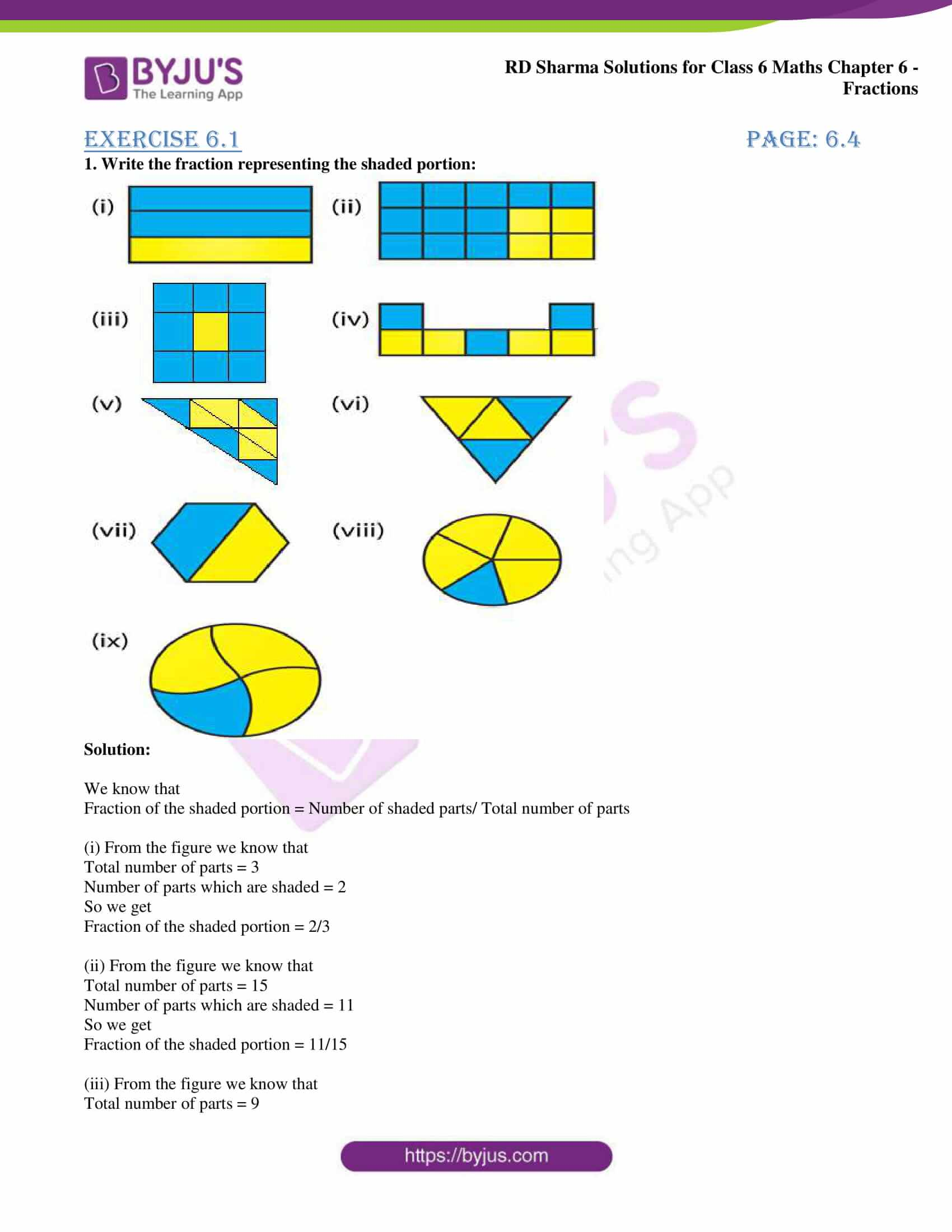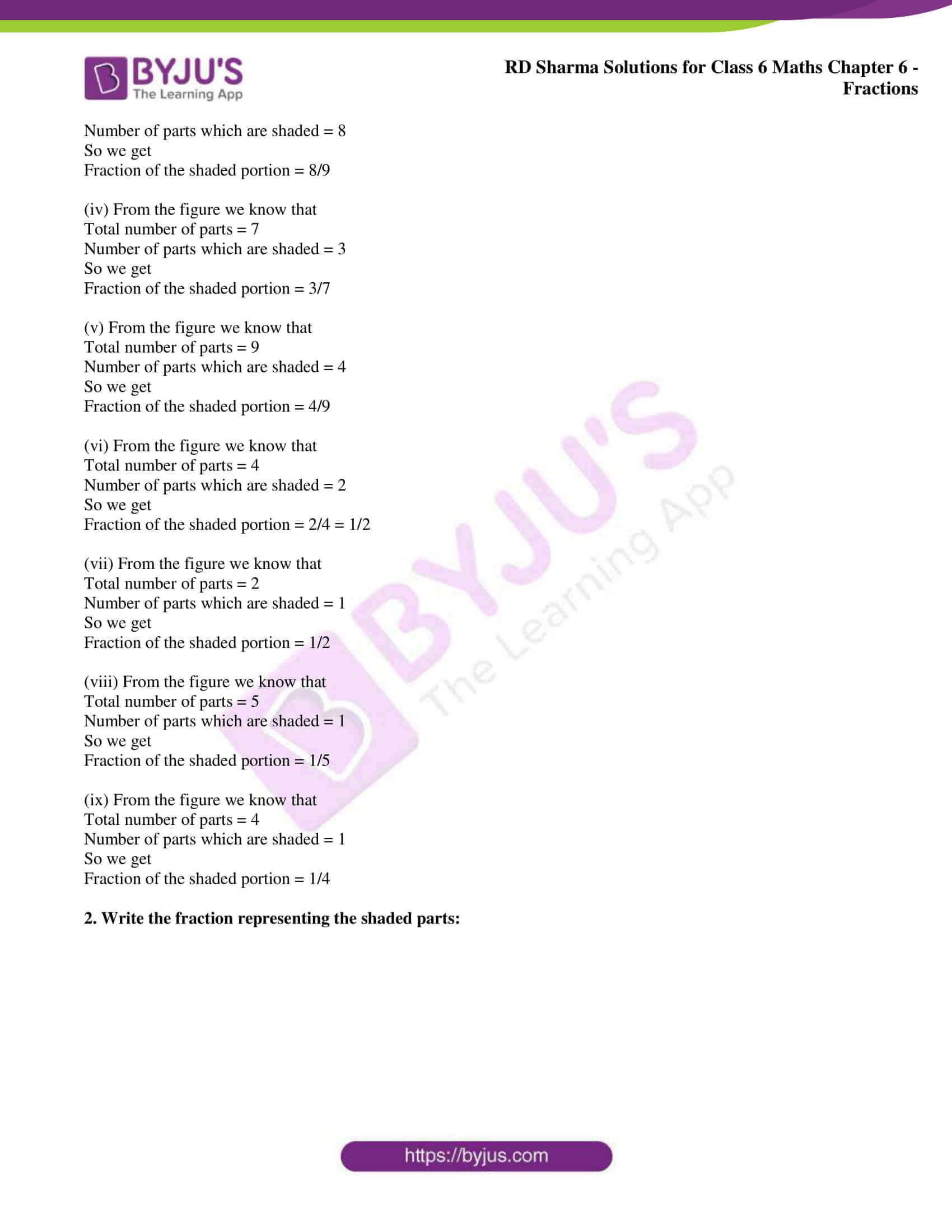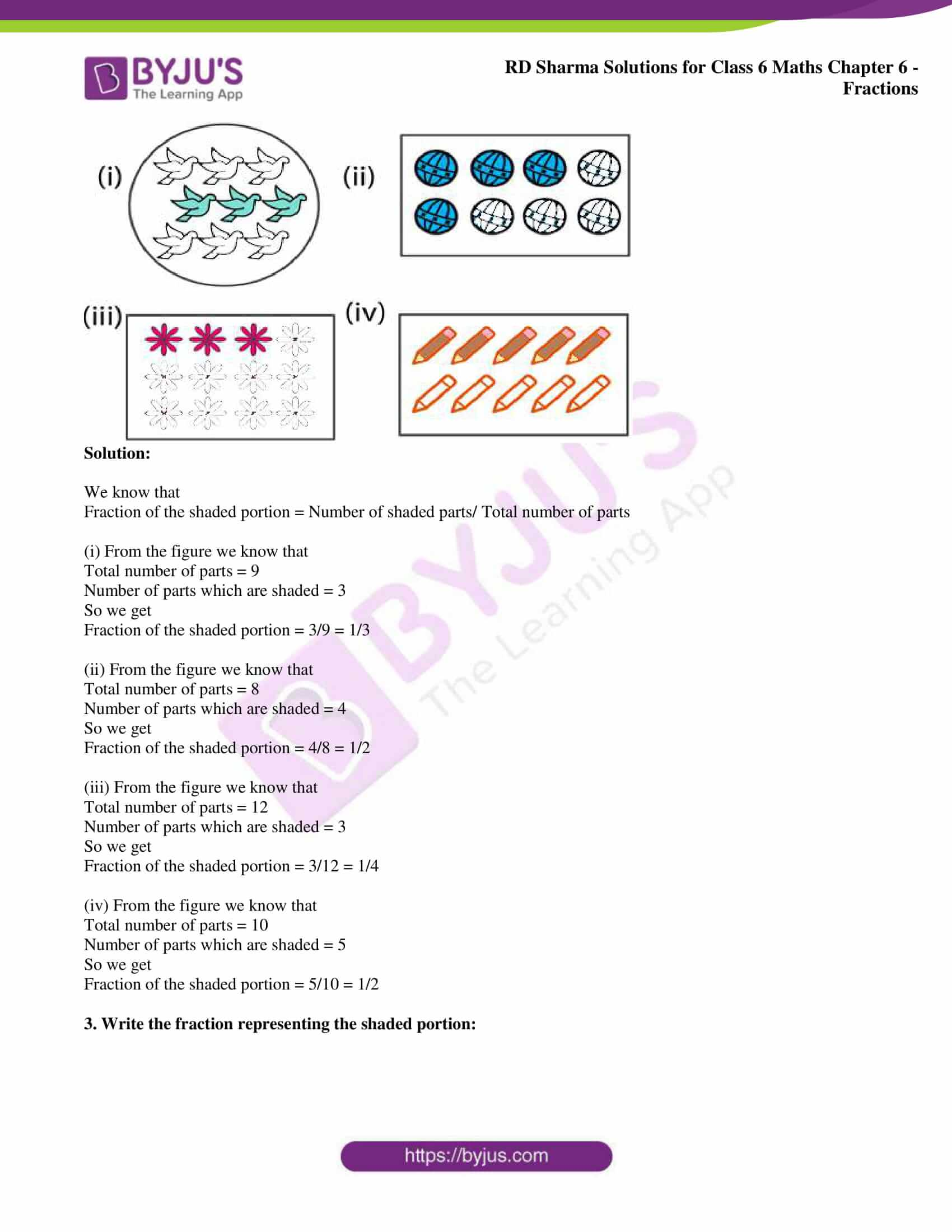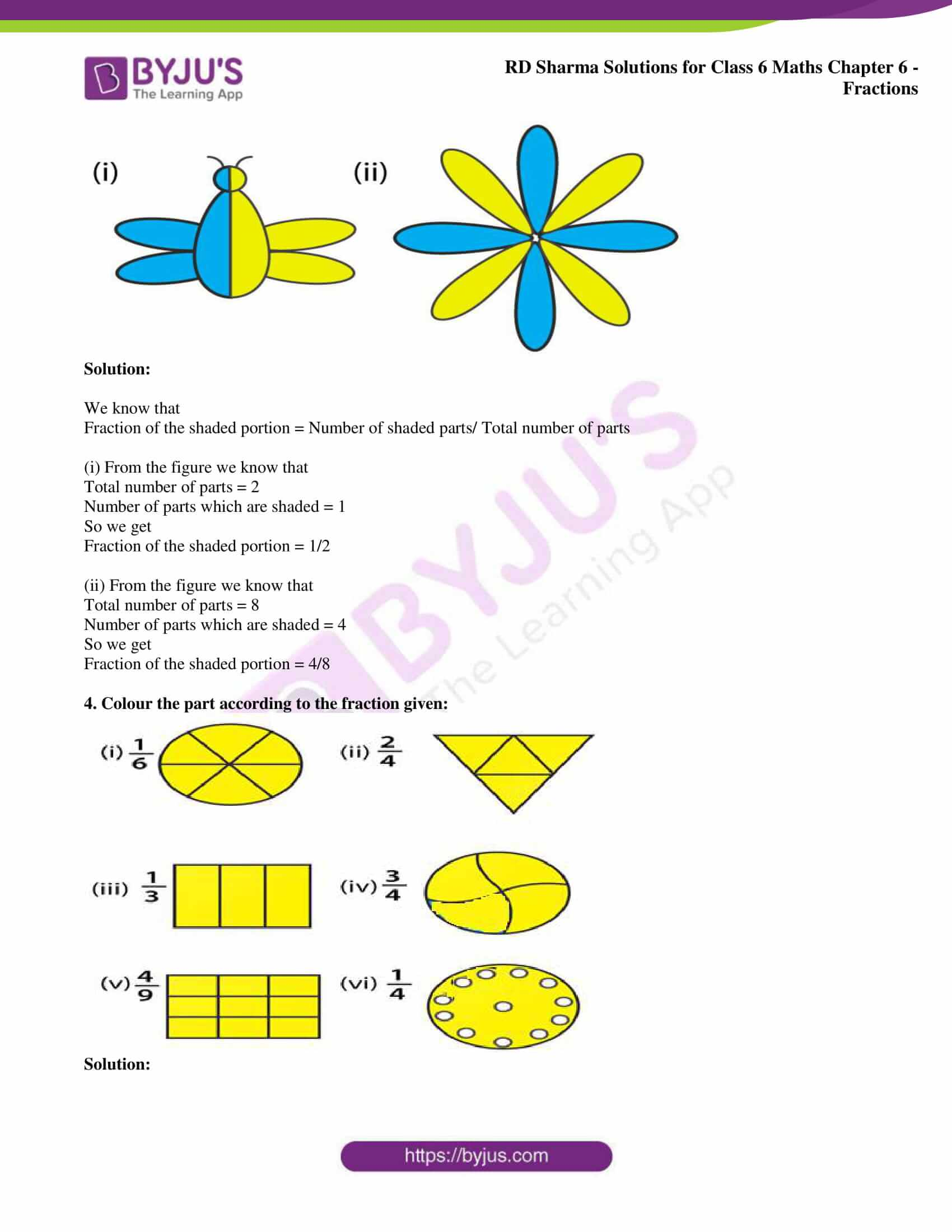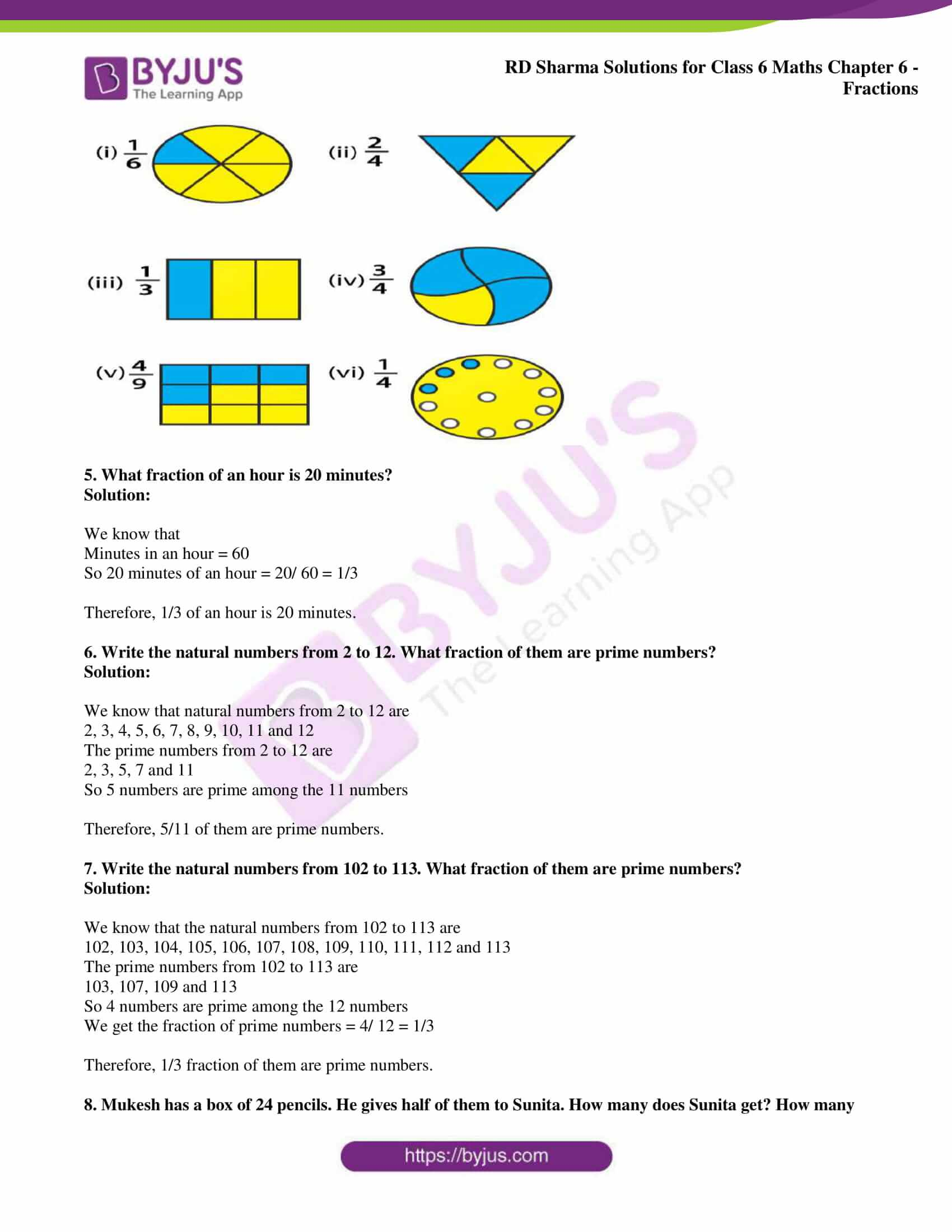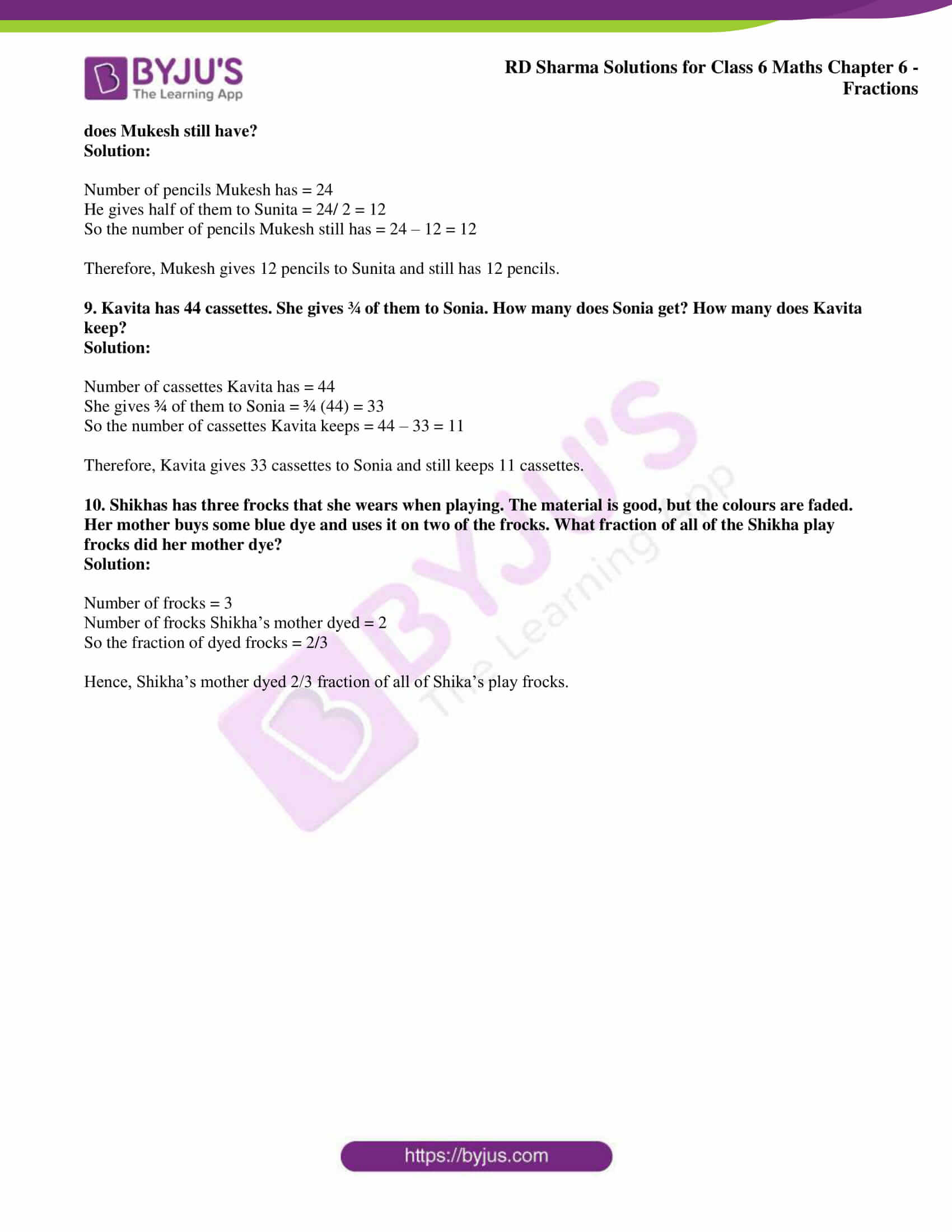### Access answers to Maths RD Sharma Solutions for Class 6 Chapter 6: Fractions Exercise 6.1

1. Write the fraction representing the shaded portion: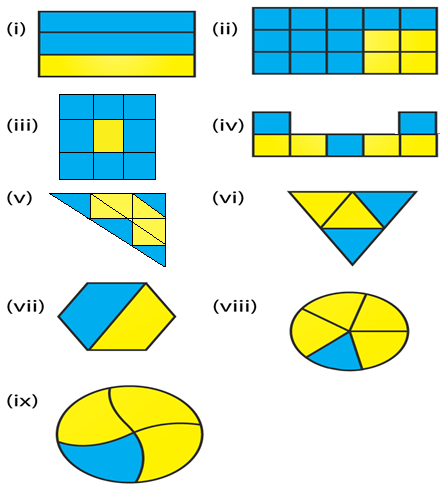Solution:

We know that

Fraction of the shaded portion = Number of shaded parts/ Total number of parts

(i) From the figure we know that

Total number of parts = 3

Number of parts which are shaded = 2

So we get

Fraction of the shaded portion = 2/3

(ii) From the figure we know that

Total number of parts = 15

Number of parts which are shaded = 11

So we get

Fraction of the shaded portion = 11/15

(iii) From the figure we know that

Total number of parts = 9

Number of parts which are shaded = 8

So we get

Fraction of the shaded portion = 8/9

(iv) From the figure we know that

Total number of parts = 7

Number of parts which are shaded = 3

So we get

Fraction of the shaded portion = 3/7

(v) From the figure we know that

Total number of parts = 9

Number of parts which are shaded = 4

So we get

Fraction of the shaded portion = 4/9

(vi) From the figure we know that

Total number of parts = 4

Number of parts which are shaded = 2

So we get

Fraction of the shaded portion = 2/4 = 1/2

(vii) From the figure we know that

Total number of parts = 2

Number of parts which are shaded = 1

So we get

Fraction of the shaded portion = 1/2

(viii) From the figure we know that

Total number of parts = 5

Number of parts which are shaded = 1

So we get

Fraction of the shaded portion = 1/5

(ix) From the figure we know that

Total number of parts = 4

Number of parts which are shaded = 1

So we get

Fraction of the shaded portion = 1/4

2. Write the fraction representing the shaded parts: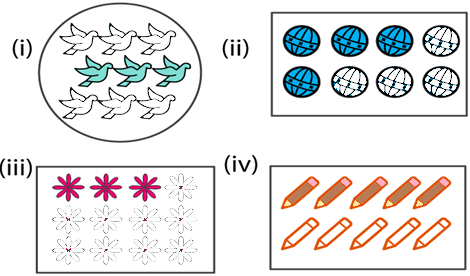Solution:

We know that

Fraction of the shaded portion = Number of shaded parts/ Total number of parts

(i) From the figure we know that

Total number of parts = 9

Number of parts which are shaded = 3

So we get

Fraction of the shaded portion = 3/9 = 1/3

(ii) From the figure we know that

Total number of parts = 8

Number of parts which are shaded = 4

So we get

Fraction of the shaded portion = 4/8 = 1/2

(iii) From the figure we know that

Total number of parts = 12

Number of parts which are shaded = 3

So we get

Fraction of the shaded portion = 3/12 = 1/4

(iv) From the figure we know that

Total number of parts = 10

Number of parts which are shaded = 5

So we get

Fraction of the shaded portion = 5/10 = 1/2

3. Write the fraction representing the shaded portion: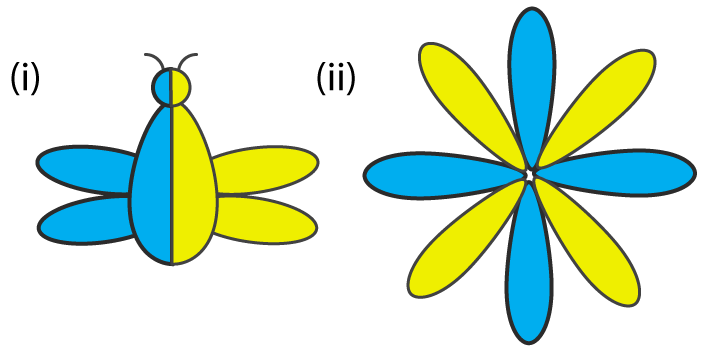Solution:

We know that

Fraction of the shaded portion = Number of shaded parts/ Total number of parts

(i) From the figure we know that

Total number of parts = 2

Number of parts which are shaded = 1

So we get

Fraction of the shaded portion = 1/2

(ii) From the figure we know that

Total number of parts = 8

Number of parts which are shaded = 4

So we get

Fraction of the shaded portion = 4/8

4. Colour the part according to the fraction given: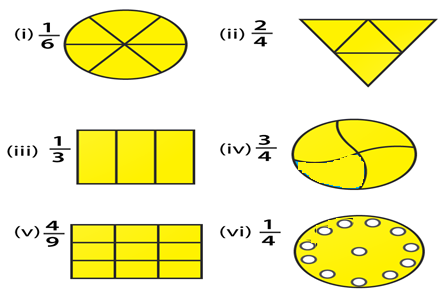Solution: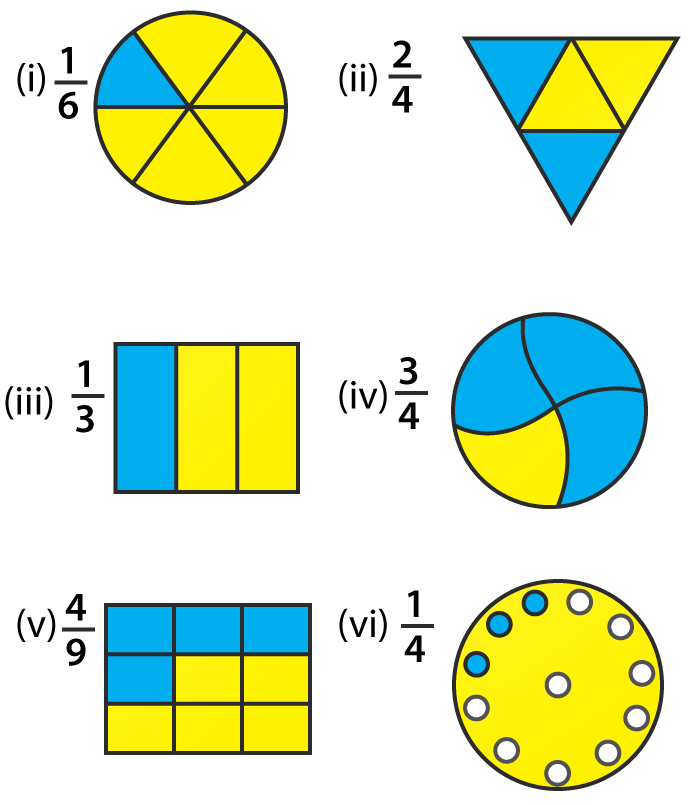5. What fraction of an hour is 20 minutes?

Solution:

We know that

Minutes in an hour = 60

So 20 minutes of an hour = 20/ 60 = 1/3

Therefore, 1/3 of an hour is 20 minutes.

6. Write the natural numbers from 2 to 12. What fraction of them are prime numbers?

Solution:

We know that natural numbers from 2 to 12 are

2, 3, 4, 5, 6, 7, 8, 9, 10, 11 and 12

The prime numbers from 2 to 12 are

2, 3, 5, 7 and 11

So 5 numbers are prime among the 11 numbers

Therefore, 5/11 of them are prime numbers.

7. Write the natural numbers from 102 to 113. What fraction of them are prime numbers?

Solution:

We know that the natural numbers from 102 to 113 are

102, 103, 104, 105, 106, 107, 108, 109, 110, 111, 112 and 113

The prime numbers from 102 to 113 are

103, 107, 109 and 113

So 4 numbers are prime among the 12 numbers

We get the fraction of prime numbers = 4/ 12 = 1/3

Therefore, 1/3 fraction of them are prime numbers.

8. Mukesh has a box of 24 pencils. He gives half of them to Sunita. How many does Sunita get? How many does Mukesh still have?

Solution:

Number of pencils Mukesh has = 24

He gives half of them to Sunita = 24/ 2 = 12

So the number of pencils Mukesh still has = 24 – 12 = 12

Therefore, Mukesh gives 12 pencils to Sunita and still has 12 pencils.

9. Kavita has 44 cassettes. She gives ¾ of them to Sonia. How many does Sonia get? How many does Kavita keep?

Solution:

Number of cassettes Kavita has = 44

She gives ¾ of them to Sonia = ¾ (44) = 33

So the number of cassettes Kavita keeps = 44 – 33 = 11

Therefore, Kavita gives 33 cassettes to Sonia and still keeps 11 cassettes.

10. Shikhas has three frocks that she wears when playing. The material is good, but the colours are faded. Her mother buys some blue dye and uses it on two of the frocks. What fraction of all of the Shikha play frocks did her mother dye?

Solution:

Number of frocks = 3

Number of frocks Shikha’s mother dyed = 2

So the fraction of dyed frocks = 2/3

Hence, Shikha’s mother dyed 2/3 fraction of all of Shika’s play frocks.